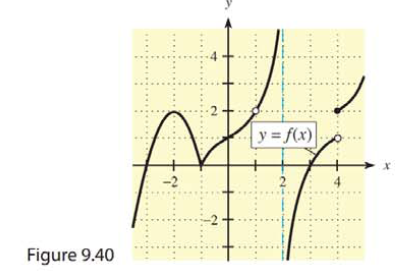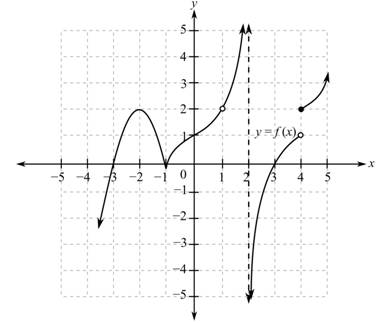Chapter 9, Problem 24RE### Mathematical Applications for the ...

11th Edition
Ronald J. Harshbarger + 1 other
ISBN: 9781305108042

#### Solutions

Chapter
Section### Mathematical Applications for the ...

11th Edition
Ronald J. Harshbarger + 1 other
ISBN: 9781305108042
Textbook Problem

# Use the graph of y = f(x) in Figure 9.40 to answer the questions in Problems 23 and 24.Is f(x) continuous at ( a ) x =   − 2 ? ( b ) x =   2 ?

(a)

To determine

Whether the function f(x) with the following graph, is continuous at x=2.Explanation

Given Information:

The graph of the function, f(x), is

Explanation:

Consider the provided graph of function,

A function is continuous at x=c, when the value of f(c) exists, the value of the limit limxcf(x) exists and limxcf(x)=f(c).

From the graph, the value of the function at x=2 is

f(2)=2

A limit limxcf(x) exists if the left-hand limit, limxcf(x), is equal to the right-hand limit, limxc+f(x).

The left hand limit represents the value to which the function approaches for all values of x, such that x<c

(b)

To determine

Whether the function f(x) with the following graph, is continuous at x=2.

The graph is,### Still sussing out bartleby?

Check out a sample textbook solution.

See a sample solution

#### The Solution to Your Study Problems

Bartleby provides explanations to thousands of textbook problems written by our experts, many with advanced degrees!

Get Started

#### Find more solutions based on key concepts